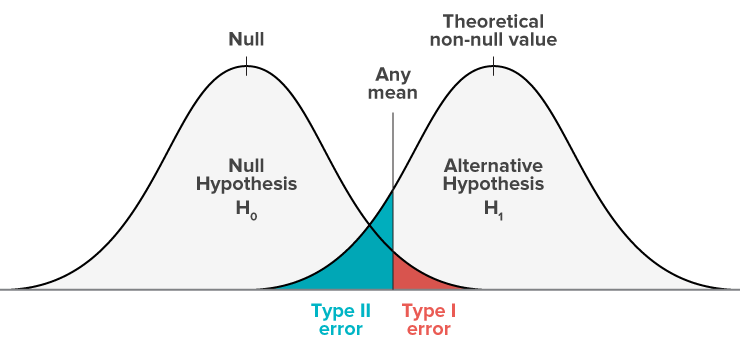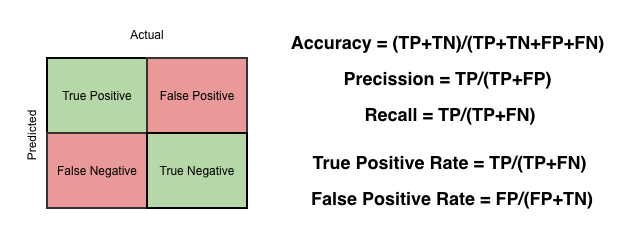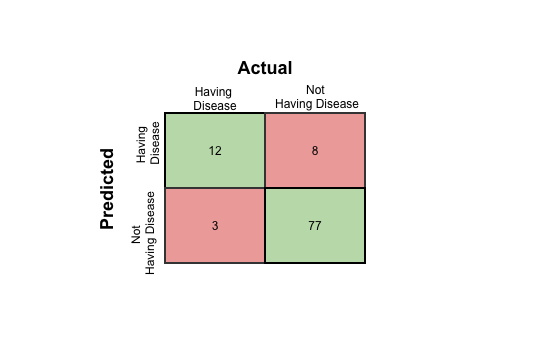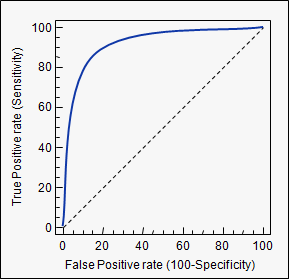In machine learning, we often use the classification models to get a predicted result of population data. Classification is one of the two sections of supervised learning, and it deals with data from different categories. The training dataset trains the model to predict the unknown labels of population data. There are multiple algorithms: Logistic regression, K-nearest neighbour, Decision tree, Naive Bayes etc. All these algorithms have their own style of execution and different techniques of prediction. To find the most suitable algorithm for a particular business problem, there are few model evaluation techniques. In this article different model evaluation techniques will be discussed.

### Confusion Matrix

It probably got its name from the state of confusion it deals with. If you remember  hypothesis testing, you may recall the two errors we defined as type-I and type-II. As depicted in Fig.1, type-I error occurs when null hypothesis is rejected, which should not be in actual.  Type-II error occurs when the alternate hypothesis is true, but you are failing to reject null hypothesis.Fig.1: Type-I and Type-II errors

In figure 1 it is depicted clearly that the choice of confidence interval affects the probabilities of these errors to occur. But if you try to reduce either of these errors, it will result in the increase of the other one.

So, what is confusion matrix?Fig.2: Confusion Matrix

Confusion matrix is the image given above. It is a matrix representation of the results of any binary testing. For example let us take the case of predicting a disease. You have done some medical testing and with the help of the results of those tests, you are going to predict whether the person is having a disease. So, actually you are going to validate if the hypothesis of declaring a person as having disease is acceptable or not. Say, among 100 people you are predicting 20 people to have the disease. In actual only 15 people to have the disease and among those 15 people you have diagnosed 12 people correctly. So, if I put the result in a confusion matrix, it will look like the following —Fig.3: Confusion Matrix of prediction a disease

So, if we compare fig.3 with fig.2 we will find —

1. True Positive: 12 (You have predicted the positive case correctly!)
2. True Negative: 77 (You have predicted negative case correctly!)
3. False Positive: 8 ( You have predicted these people as having a disease, which they acutally don’t. But do not worry, this can be rectified in further medical analysis. So, this is a low risk error. This is type-II error in this case.)
4. False Negative: 3 (Oh ho! You have predicted these three poor fellows as fit. But actually they have the disease. This is dangerous! Be careful! This is type-I error in this case.)

Now if I ask what is the accuracy of the prediction model what I followed to get these results, the answer should be the ratio of the accurately predicted number and the total number of people which is (12+77)/100 = 0.89. If you study the confusion matrix thoroughly you will find the following things —

1. The top row is depicting the total number of predictions you did as having the disease. Among these predictions, you have predicted 12 people correctly to have the disease in actual. So, the ratio, 12/(12+8) = 0.6 is the measure of the accuracy of your model in detecting a person to have the disease. This is called Precision of the model.
2. Now, take the first column. This column represents the total number of people who are having the disease in actual. And you have predicted correctly for 12 of them. So, the ratio, 12/(12+3) = 0.8 is the measure of the accuracy of your model to detect a person having disease out of all the people who are having the disease in actual. This is termed as Recall.

Now, you may ask the question that why do we need to measure precision or recall to evaluate the model?

The answer is it is highly recommended when a particular result is very sensitive. For example you are going to build a model for a bank to predict fraudulent transactions. It is not very common to have a fraudulent transaction. In 1000 transactions, there may be 1 transaction which is fraud. So, undoubtedly your model will predict a transaction as non-fraudulent very accurately. So, in this case the whole accuracy does not matter as it will be always very high irrespective of the accuracy of the prediction of the fraudulent transactions as that is of very low percentage in the whole population. But the prediction of a fraudulent transaction as non-fraudulent is not desirable. So, in this case the measurement of precision will take a vital role to evaluate the model. It will help to understand out of all the actual fraudulent transactions, how many are being predicted. If it is low, even if the overall accuracy if high, the model is not acceptable.

### Receiver Operating Characteristics (ROC) Curve

Measuring the area under the ROC curve is also a very useful method for evaluating a model. ROC is the ratio of True Positive Rate (TPR) and False Positive Rate (FPR) (see fig.2). In our disease detection example, TPR is the measure of the ratio between the number of accurate predictions of people having the disease and the total number of people having the disease in actual. FPR is the ratio between the number of people who are predicted as not to have disease correctly and the total number of people who are not having the disease in actual. So, if we plot the curve, it comes like this —Fig.4: ROC curve (source: https://www.medcalc.org/manual/roc-curves.php)

The blue line denotes the change of TPR with different FPR for a model. More the ratio of the area under the curve and the total area (100 x 100 in this case) defines more the accuracy of the model. If it becomes 1, the model will be overfit and if it is equal below 0.5 (i.e when the curve is along the dotted diagonal line), the model will be too inaccurate to use.

For classification models, there are many other evaluation methods like Gain and Lift charts, Gini coefficient etc. But the in-depth knowledge about the confusion matrix can help to evaluate any classification model very effectively. So, in this article I tried to demystify the confusions around the confusion matrix to help the readers.

Happy modelling!

Saikat Bhattacharya is a Senior Software engineer at Freshworks, and is pursuing the PGP-Machine Learning program from Great Learning. This article originally appeared on Towards Data Science and has been syndicated with permission from the author.

0